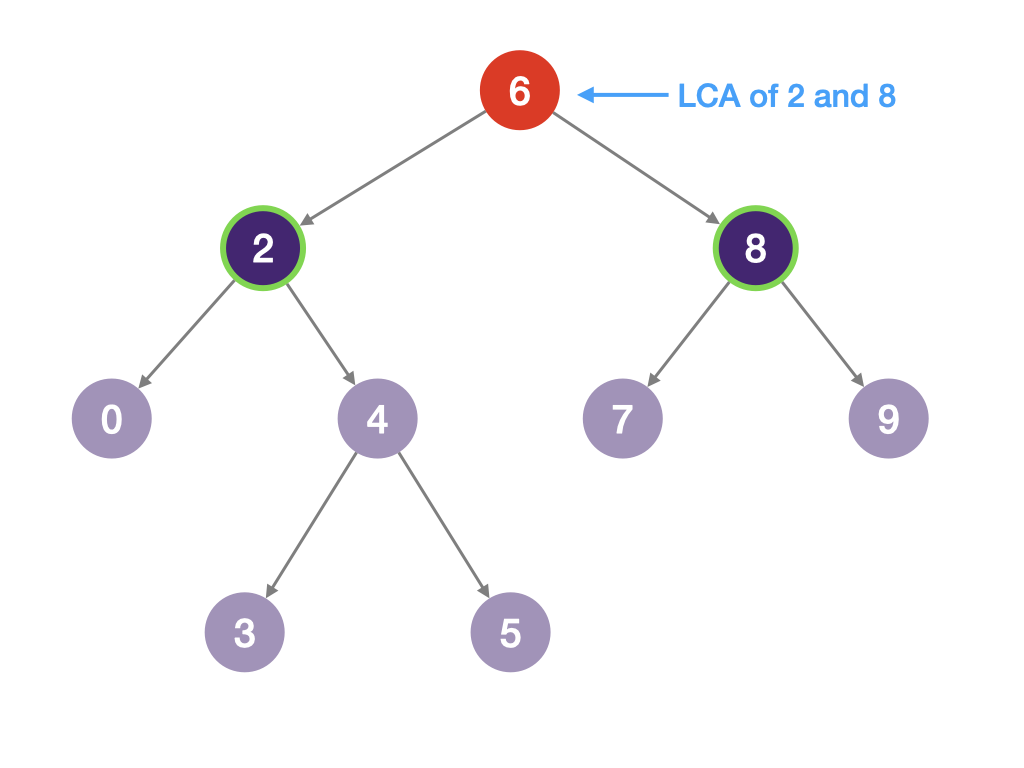# Lowest Common Ancestor of a Binary Search Tree

Given a binary search tree (BST), find the lowest common ancestor (LCA) node of two given nodes in the BST.

According to the definition of LCA on Wikipedia: “The lowest common ancestor is defined between two nodes `p` and `q` as the lowest node in `T` that has both `p` and `q` as descendants (where we allow a node to be a descendant of itself).”

### Input

• `bst`: a binary tree representing the existing BST.
• `p`: the value of node p as described in the question
• `q`: the value of node q as described in the question

### Output

The value of the LCA between nodes p and q

### Examples

#### Example 1:

Input:

``````1bst = [6,2,8,0,4,7,9,x,x,3,5]
2p = 2
3q = 8``````

Output: `6`

Explanation:The ancestors of node `p` with value 2 are node 2 and node 6. The ancestors of node `q` with value 8 are node 8 and node 6.

The lowest common ancestors between these two nodes is 6.